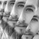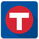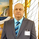2772 просмотров
This is an experimental study designed using data from Bollinger Bands to determine price squeeze ranges and active levels of support and resistance .
First, a set of Bollinger Bands using a Coefficient of Variation weighted moving average as the basis is calculated.
Then, the relative percentage of current bandwidth to maximum bandwidth over the specified sampling period determines the relative squeeze.
The box is outlined by drawing the current highest and lowest source value over the sampling period whenever a squeeze is active.
I've included the COVWMA in the visualization for additional confirmation of price activity.

Custom Bar color scheme is included.
Added alternate moving average types. The available moving averages now in this script are:
-Exponential Moving Average
-Simple Moving Average
-Smoothed Moving Average
-Weighted Moving Average
-Volume Weighted Moving Average
-Least Squares Moving Average
-Arnaud Legoux Moving Average
-Hull Moving Average
-Coefficient of Variation Weighted Moving Average

Refined the color scheme for both bar colors and the moving averages.

Please note: Each moving average type generates different box values, so play around with them and find the one that works best for you.

## Комментарии//@version=4
//by Donovan Wall

//This is an experimental study designed using data from Bollinger Bands to determine price squeeze ranges and active levels of support and resistance.
//First, a set of Bollinger Bands using a Coefficient of Variation weighted moving average as the basis is calculated.
//Then, the relative percentage of current bandwidth to maximum bandwidth over the specified sampling period determines the relative squeeze.
//The box is outlined by drawing the current highest and lowest source value over the sampling period whenever a squeeze is active.
//I've included the COVWMA in the visualization for additional confirmation of price activity.

//Custom Bar color scheme is included.

//---------------------------------------------------------------------------------------------------------------------------------------------------------------
//Added alternate moving average types. The available moving averages now in this script are:
// -Exponential Moving Average
// -Simple Moving Average
// -Smoothed Moving Average
// -Weighted Moving Average
// -Volume Weighted Moving Average
// -Least Squares Moving Average
// -Arnaud Legoux Moving Average
// -Hull Moving Average
// -Coefficient of Variation Weighted Moving Average

//Refined the color scheme for both bar colors and the moving averages.

//Please note: Each moving average type generates different box values, so play around with them and find the one that works best for you.

//-----------------------------------------------------------------------------------------------------------------------------------------------------------------
//Inputs
//-----------------------------------------------------------------------------------------------------------------------------------------------------------------

//Source
src = input(defval=hlc3, title="Source")

//Periods
per = input(defval=21, minval=1, title="Sampling Period")

//MA Type
matype = input(defval="COVWMA", title="MA Type (Available Inputs: 'EMA', 'SMA', 'SMMA', 'WMA', 'VWMA', 'LSMA', 'ALMA', 'HULLMA', 'COVWMA', 'FRAMA', 'KAMA')")

//Standard Deviations
ndev = input(defval=2, minval=1, title="Number of Deviations for Squeeze Calculation")

//Relative Squeeze Threshold
sr = input(defval=50, minval=0, maxval=100, step=0.01, title="Relative Squeeze % Threshold")

//LSMA Offset
loff = input(defval=0, minval=0, title="Offset (if LSMA)")

//ALMA Offset and Sigma
aoff = input(defval=0.85, step=0.01, minval=0, title="Offset (if ALMA)")
sigma = input(defval=6, minval=0, title="Sigma (if ALMA)")

//FRAMA Coefficient
w = input(defval=-4.6, title="Coefficient (if FRAMA)")

//KAMA Smoothing Constant
fast = input(defval=0.666, step=0.001, title="Smoothing Constant Fast End (if KAMA)")
slow = input(defval=0.0645, step=0.0001, title="Smoothing Constant S
Ответитьrichhubb
@richhubb, Thanks for taking the time to update this to v4! I'm going to look it over and publish it with some other updates and new tools I have in the works.
ОтветитьDonovanWall
@DonovanWall, This is one of my favorite studies on TradingView Thanks Donovan! A nice addition to this study(or possibly a new study) would be % price change above/below last support/resistance level breakthrough to warn/alert of possible consolidation areas or areas of potential price reversal Thanks again for an incredibly insightful study Or maybe you have a study that replicates something similar Perhaps your Z Score indicator?
ОтветитьWhat are we calling A and B on the function here? Thanks.
Ответить